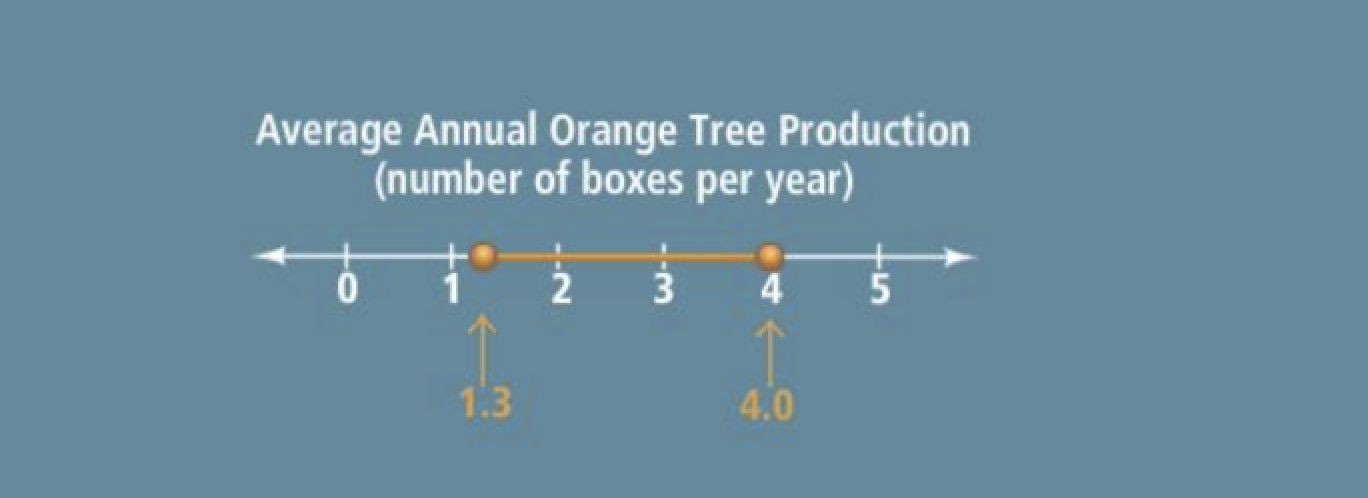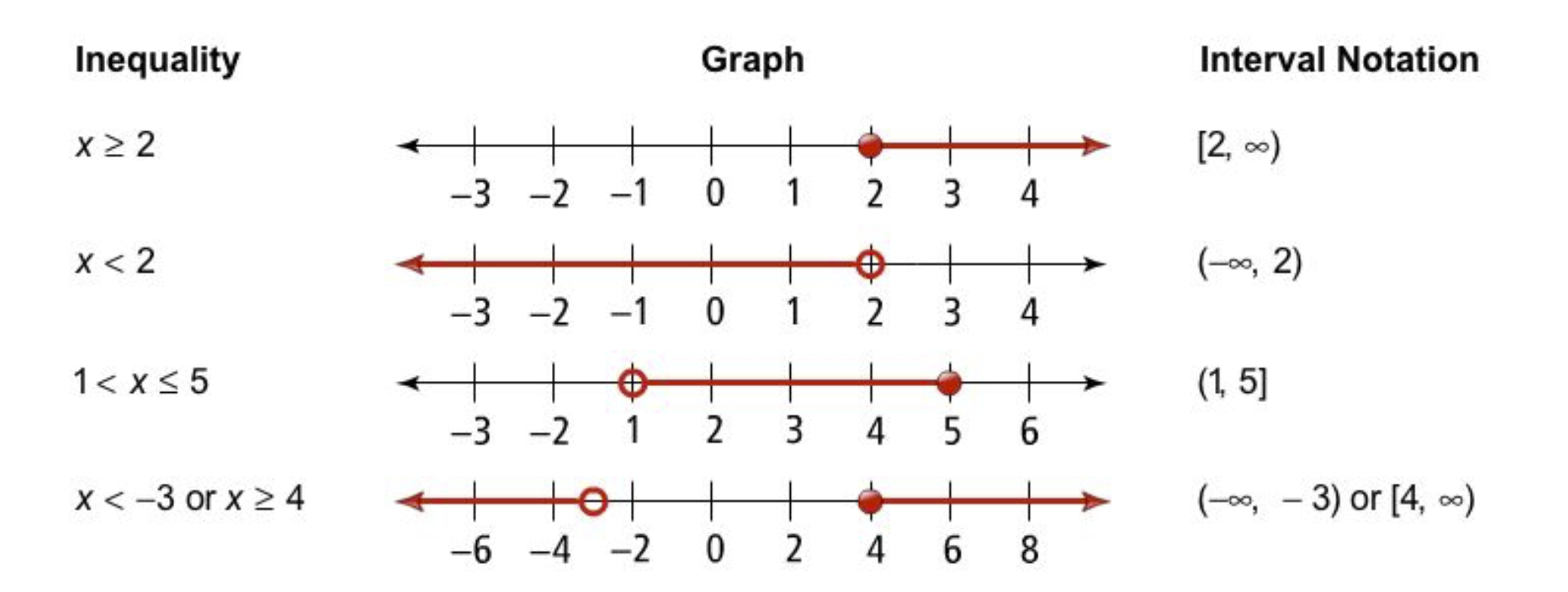Algebra 1 3-6 Guided Practice: Compound Inequalities
starstarstarstarstarstarstarstarstarstar
by Matthew Richardson
| 12 Questions1
1
10
Solve It! The diagram shows the number of boxes of oranges that an orange tree can produce in 1 year. An orange grower earns \$9.50 for each box of oranges that he sells. How much could the grower expect to earn in 1 year from 1 tree? Explain your reasoning.2
5
Problem 1 Got It? Write a compound inequality that represents the phrase.

all real numbers that are greater than or equal to -4 and less than 6
4 < x ≤ 6
-6 ≤ x < 4
6 < x ≤ -4
-4 ≤ x < 6
3
10
Problem 1 Got It? Graph the compound inequality that represents the phrase.

all real numbers that are greater than or equal to -4 and less than 6
4
5
Problem 1 Got It? Write a compound inequality that represents the phrase.

all real numbers that are less than or equal to 2.5 or greater than 6
x ≤ 2.5 or x > 6
x ≤ 6 or x > 2.5
6 ≤ x < 2.5
x ≤ -2.5 or x > 6
5
10
Problem 1 Got It? Graph the compound inequality that represents the phrase.

all real numbers that are less than or equal to 2.5 or greater than 6
6
10
Problem 1 Got It? What is the difference between "x is between -5 and 7" and "x is between -5 and 7, inclusive"?
7
10
Problem 2 Got It? What are the solutions of -2 < 3y - 4 < 14? Graph the solutions.

Remember to write the simplified compound inequality before graphing the solutions on the number line.
8
10
Problem 3 Got It? Reasoning: Suppose you scored 78, 78, and 79 on the first three tests. Is it possible for you to earn a B in the course? Explain.

Recall that in this scenario Bs represent grades of 84 - 86, inclusive.
Assume that 100 is the maximum grade that you can earn in the course.
9
10
Problem 4 Got It? What are the solutions of -2y + 7 < 1 or 4y + 3 ≤ -5? Graph the solutions.

Remember to write the simplified compound inequality before graphing the solutions on the number line.10
10
Problem 5 Got It? How do you write (-2, 7] as an inequality?
-2 < b ≤ 7
-2 ≤ b < 7
-2 > b ≥ 7
7 < b ≤ -2
11
10
Problem 5 Got It? What is the graph of (-2, 7]?
12
10
Problem 5 Got It? How do you write y > 7 in interval notation?
[7, ∞)
(7, ∞]
[7, ∞]
(7, ∞)